# What Is The Ceiling Function? (3 Key Things To Remember)

The ceiling function takes a set of real numbers and returns whole numbers, without any fractions or decimals.  However, it is a good idea to know exactly how it works.

So, what is the ceiling function?  The ceiling function ceil(x) (or x⌉) takes a real number x as input and returns an integer y as output. The output y is the least integer greater than or equal to x. That is, y = ceil(x) is the integer where y >= x and y – 1 < x. For example, ceil(1.3) = 2, ceil(1.7) = 2, and ceil(2) = 2.

Of course, for negative values of x (x < 0), taking the ceiling of x is the same as truncating x (cutting off the decimal part).

In this article, we will talk about what the ceiling function is, what it looks like on a graph, and some of the variations of the graph.  We’ll also answer some common questions about the ceiling function.

Let’s get started.

## What Is The Ceiling Function?

The ceiling function ceil(x) is also called the least integer function.  It is sometimes denoted by the ceiling function symbol ⌈x⌉.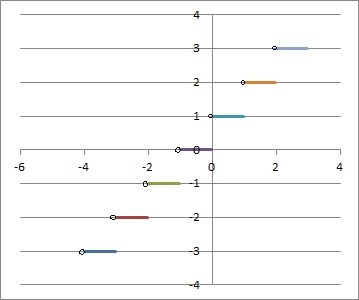Here we have the graph of the ceiling function, ceil(x) or ⌈x⌉.

The ceiling function y = ceil(x) takes a real number x as input (so the domain is the set of all real numbers).

The output y of the ceiling function is an integer y.  The output y is the least integer greater than or equal to x.

Learn Siemens Tia Portal Programmin...
Learn Siemens Tia Portal Programming Online - CEIL, FLOOR, TRUNC

In other words, y is the unique integer that satisfies the equation y – 1 < x <= y.

The ceiling function behaves a little differently depending on whether x is negative or not:

• For nonnegative values of x (x >= 0), ceil(x) – 1 = trunc(x).  Alternatively, ceil(x) = trunc(x) for x >= 0.
• For negative values of x, ceil(x) = trunc(x) is the same as truncating x (removing the decimal or fraction part).

### What Does The Ceiling Function Do?

The ceiling function takes a real number (which may contain decimals or fractions) as input and returns an integer (whole number) without decimals or fractions as the output.

In other words, ceil(x) finds the closest integer to x that is greater than or equal to x.  For a given real number x, there are infinitely many integers greater than or equal to x, but ceil(x) is the smallest one of those.

### How Do You Find The Ceiling Of A Number?

Here are the steps to find the ceiling of a number x:

• If x is an integer (a whole number with no fractions or decimals), then ceil(x) = x.
• If x is not an integer, look at the sign of x (positive or negative).
• If x is negative, then truncate x (cut off the decimal or fraction part) to get ceil(x).
• If x is positive, then truncate x and add 1 to get ceil (x).

#### Example 1: Ceiling Of 6

Since 6 is an integer, ceil(6) = 6.

#### Example 2: Ceiling Of 4.5

Since 4.5 is not an integer, we look at the sign, which is positive.

Then we truncate 4.5 to 4.

Then we add 1 to get 5.

So ceil(4.5) = 5.

#### Example 3: Ceiling Of -2.5

Since -2.5 is not an integer, we look at the sign, which is negative.

Then we truncate -2.5 to -2.

So ceil(-2.5) = -2.

### Ceiling Function Formula

We can write a formula for the ceiling function in a couple of different ways.

One way to write ceil(x) is by using the truncate function.  There is one part for x >= 0 and one part for x < 0:

• ceil(x) = {truncate(x), x < 0 or x a nonnegative integer; truncate(x) + 1, x >= 0 and x not an integer}

Another way to write ceil(x) is by using integers:

• For each integer n, ceil(x) = n + 1 for n < x <= n + 1.

### Ceiling Function Graph

Here is the graph of the ceiling function, y = ceil(x) or y = ⌈x⌉.The ceiling function, graphed in Excel, looks somewhat like a staircase.

Note that the graph looks somewhat like a staircase.

Also, note that the graph has a jump discontinuity at every integer value of x (x = … -2, -1, 0, 1, 2, …)

Finally, observe that the y-values of the ceiling function are all integers (no fractions or decimals).

### Ceiling Function Examples

Here are some examples of ceiling functions and modifications based scaling and shifts (horizontal and vertical).

#### Example 1: Ceiling Function With A Scale

Consider the scaled ceiling function f(x) = 2*ceil(x).

This looks like the regular ceiling function (graphed earlier), but it is twice as tall (every y-value is doubled from the basic ceiling function).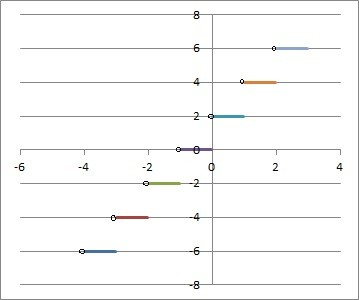This is the graph of the scaled ceiling function f(x) = 2*ceil(x).

#### Example 2: Ceiling Function With A Vertical Shift

Consider the Ceiling function f(x) = ceil(x) + 3

This looks like the regular ceiling function (graphed earlier), but it has vertical shift: it is moved up 3 units from the basic ceiling function.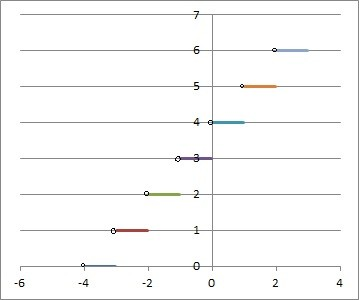This is the graph of the ceiling function with a vertical shift of 3 units up: f(x) = ceil(x) + 3.

#### Example 3: Ceiling Function With A Horizontal Shift

Consider the ceiling function f(x) = ceiling(x – 4)

This looks like the regular ceiling function (graphed earlier), but it has horizontal shift: it is moved right 4 units from the basic ceiling function.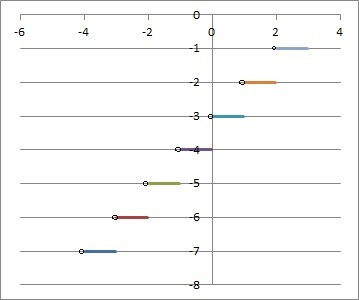This is the graph of the ceiling function with a horizontal shift of 4 units to the right: f(x) = ceil(x – 4).

#### Example 4: Ceiling Function With Scaling & Shifts

Consider the ceiling function f(x) = 2*ceiling(x – 4) + 3

This looks like the regular ceiling function (graphed earlier), but it has 3 aspects that change it:

• A horizontal shift of 4 units to the right.
• A scaling (doubled), giving it twice the height.
• A vertical shift of 3 units up.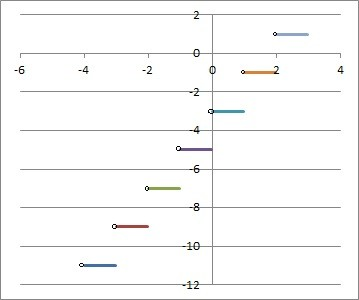This is the ceiling function f(x) = 2*ceil(x – 4) + 3.

### Is The Ceiling Function Injective (One-To-One)?

The Ceiling function is not injective, since there are always multiple x-values (inputs) that lead to the same output (y-value).

For example, for a given output value of y (an integer), both of the inputs y – 0.1 and y – 0.2 have a Ceiling of y, which means that Ceiling(y – 0.1) = y and Ceiling(y – 0.2) = y.

Thus, Ceiling(x) is not an injective function.

### Is The Ceiling Function Surjective (Onto)?

The Ceiling function Ceiling(x) is not surjective onto the set of real numbers.  Remember that the outputs of the basic Ceiling function are only integers (whole numbers with no fractions or decimals).

The Ceiling function is surjective onto the set of integers.

### Is The Ceiling Function Invertible?

The Ceiling function is not invertible, since it is not one-to-one or onto.

### Is The Ceiling Function Continuous?

The Ceiling function is not continuous, since it has a discontinuity at every integer value of x.

However, Ceiling(x) is continuous on every interval between integers.  That is, Ceiling(x) is continuous on the interval (n, n+1) for each integer n.

### Is The Ceiling Function Differentiable?

The Ceiling function is not differentiable, since it has a discontinuity at every integer value of x (and a function must be continuous to be differentiable).

However, Ceiling(x) is differentiable on every interval between integers.  The derivative of Ceiling(x) is 0 on the interval (n, n+1) for each integer n.

### Is The Ceiling Function Even Or Odd?

The Ceiling function is neither even nor odd.  Here is the proof:

Take x = 1.5.  Then:

• Ceiling(x) = Ceiling(1.5) = 2
• Ceiling(-x) = Ceiling(-1.5) = -1
• -Ceiling(x) = -Ceiling(1.5) = -2

Since Ceiling(-x) is not equal to Ceiling(x), the Ceiling function is not even.

Since Ceiling(-x) is not equal to -Ceiling(x), the Ceiling function is not odd.

### What Is The Ceiling Function In Excel?

The Ceiling function in Excel is given by CEILING, and it has 2 inputs:

• The first input is a number or a cell that contains a number.
• The second input is a significance level.  To get an integer output, use 1 for this input.

So, to find the Ceiling of the number x in Excel, use the formula

• =CEILING(x, 1)

If x in in cell A1, we would use the formula

• =CEILING(A1, 1)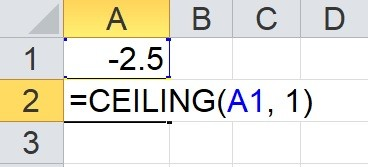The formula =CEILING(A1, 1) in cell A2 returns the ceiling of -2.5, which is -2.

### Ceiling vs Truncate

Remember that the definitions are as follows:

• The Ceiling function returns the least integer than is greater than or equal to x.
• The truncate function cuts off the decimal or fraction part of a number x, leaving only the integer part.

For negative values of x (x < 0), the Ceiling and truncate functions will return the same value.  In other words, Ceiling(x) = trunc(x) for x < 0.

For nonnegative values of x (x <= 0), there are two cases:

• Case 1: x is an integer: in this case, the Ceiling and truncate functions are the same.
• Case 2: x is not an integer: in this case, the Ceiling and truncate functions are one unit apart (ceiling is larger).  In other words, Ceiling(x) = trunc(x) + 1 when x is nonnegative and not an integer.

The following table shows some values of x, along with the outputs for both the Ceiling and truncate functions.

## Conclusion

Now you know what the ceiling function is, how it works, and what it looks like on a graph.  You also know the answers to some common questions about ceiling functions.

You can learn about the floor function here.

I hope you found this article helpful.  If so, please share it with someone who can use the information.